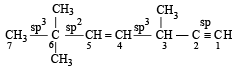Courses

# Test: Organic Chemistry - Some Basic Principles And Techniques 2 - From Past 28 Years Questions

## 30 Questions MCQ Test Chemistry 28 Years Past year papers for NEET/AIPMT Class 11 | Test: Organic Chemistry - Some Basic Principles And Techniques 2 - From Past 28 Years Questions

Description
This mock test of Test: Organic Chemistry - Some Basic Principles And Techniques 2 - From Past 28 Years Questions for NEET helps you for every NEET entrance exam. This contains 30 Multiple Choice Questions for NEET Test: Organic Chemistry - Some Basic Principles And Techniques 2 - From Past 28 Years Questions (mcq) to study with solutions a complete question bank. The solved questions answers in this Test: Organic Chemistry - Some Basic Principles And Techniques 2 - From Past 28 Years Questions quiz give you a good mix of easy questions and tough questions. NEET students definitely take this Test: Organic Chemistry - Some Basic Principles And Techniques 2 - From Past 28 Years Questions exercise for a better result in the exam. You can find other Test: Organic Chemistry - Some Basic Principles And Techniques 2 - From Past 28 Years Questions extra questions, long questions & short questions for NEET on EduRev as well by searching above.
QUESTION: 1

### Name of the compound given below is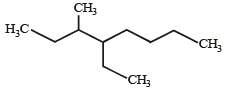Solution: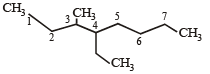4 ethyl - 3 methyl octane

QUESTION: 2

### The correct order of reactivity towards the electrophilic substitution of the compounds aniline (I), benzene (II) and nitrobenzene (III) is

Solution:

Amino group is ring activating while nitro group is deactivating. Hence, correct order is  aniline > benzene > nitrobenzene.
I > II > III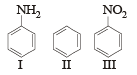–NO2 is an electron attracting group hence decrease the electron density on ring, whereas –NH2 group is electron releasing group hence increases electron density on ring. Benzene is also rich due to delocalization of electrons.

QUESTION: 3

### Which one of the following is a free-radical substitution reaction? 

Solution:

In the presence of UV rays or energy, by boiling chlorine, free radical is generated which attack the methyl carbon atom of the toluene.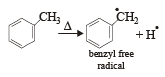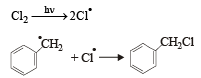QUESTION: 4

Which of the following pairs of compounds are enantiomers? 

Solution:

Compound which are mirror image of each other and are not superimposable are termed as enantiomers.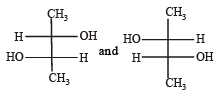are enantiomers

QUESTION: 5

Number of chiral carbons in β - D - (+) - glucose is 

Solution: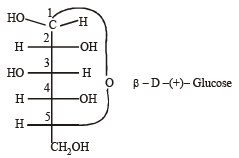Carbon atoms from C1 to C5 are chiral

QUESTION: 6

Which of the following is least reactive in a nucleophilic substitution r eaction. 

Solution:

H2C = CHCl is capable of showing resonance which develops a partial double bond, character to C–Cl bond, thereby making it less reactive toward  nucleophilic substitution. ..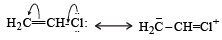QUESTION: 7

The molecular formula of diphenyl methane,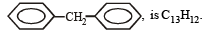How many structural isomers are possible when one of the hydrogens is replaced by a chlorine atom?

Solution:

In diphenylmethane monochlorination at following positions will produce structured isomers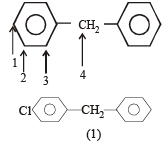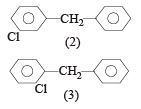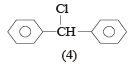QUESTION: 8

The chirality of the compound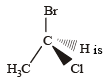Solution:

Clock wise rotation.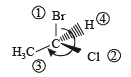Hence configuration is R.
If the eye travel in a clockwise direction, the configuration is specified as the order of priority is Br > Cl > CH3 > H

QUESTION: 9

Which one of the following pairs represen ts stereoisomerism? 

Solution:

Optical and geometrical isomerism pair up to exhibit stereoisomerism. This is because the isomers differ only in their orientation in space.

QUESTION: 10

Which of the following undergoes nucleophilic substitution exclusively by SN1 mechanism? 

Solution:

SN1 reaction is favour ed by heavy gr oups on the carbon atom attached to halogen i.e Benzyl > allyl > tertiary > primary > secondary > primary > alkyl halides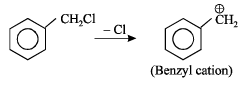Obtained from SN1 path.
This molecule is resonance stabilised.

QUESTION: 11

Which amongst the following is the most stable carbocation? 

Solution:

More the number of alkyl groups, the greater the dispersal of positive charge and therefore more the stability of carbocation hence.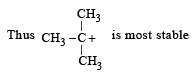QUESTION: 12

Which one of the following compounds is most acidic? 

Solution:

Phenols are more acidic than alcohol as they are resonance stabilised whereas alcohols are not .
Further nitro is an electron withdrawing which increases acidic character and facilitates release of proton, whereas –CH3 is an electron donating group.Which decreases acidic character , thus removal of H+ becomes very difficult.

QUESTION: 13

Names of some compounds are given. Which one is not correct in IUPAC system? 

Solution:

Correct IUPAC name of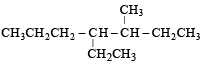is 4-Ethyl-3–methyl heptane

QUESTION: 14

The best method for the separation of naphthalene and benzoic acid from their mixture is: [2 00 5]

Solution:

Among the given compounds naph thelene is volatile but benzoic acid is non-volatile (it forms a dimer). So, the best method for their separation is sublimation, which is applicable to compounds which can be converted directly into the vapour phase from its solid state on heating and back to the solid state on cooling. Hence it is the most appropriate method.

QUESTION: 15

The general molecular formula, which represents the homologous series of alkanols is 

Solution:

General molecular formula of alkanols is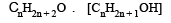QUESTION: 16

The IUPAC name of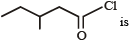Solution: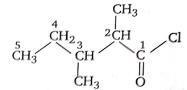It is 2, 3 dimethyl pentanoyl chloride.

QUESTION: 17

The correct order regarding the electronegativity of hybrid orbitals of carbon is 

Solution:

Among the three given hybrid orbitals, sp hybrid orbital is most electronegative.
Contribution of s in sp hybrid orbital is maximum (50%) so this orbital is closer to nucleus. Naturally it will have greater tendency to pull electron towards it. Hence it becomes more electronegative and sp3 becomes least electronegative as it have only 25% S character.

QUESTION: 18

Which of the following is not chiral ? 

Solution: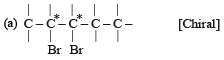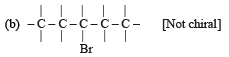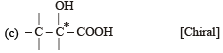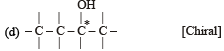* marked are chiral carbons.

QUESTION: 19

Consider the following compounds. 

C6H5COCl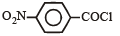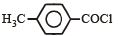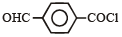The correct decreasing order of their reactivitytowards hydrolysis is

Solution:

The degree of hydrolysis increases as the magnitude of positive charge on carbonyl group increases. Electron withdrawing group increases the positive charge and electron releasing group decreases the positive charge. Among these NO2 & CHO are electron withdrawing group from which NO2 has more –I effect than –CHO. On the other hand CH3 is a electron releasing group therefore the order of reactivity towards hydrolysis is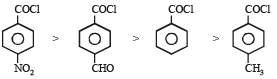QUESTION: 20

If there is no rotation of plane polarised light by a compound in a specific solvent, though to be chiral, it may mean that 

Solution:

Compounds which do not show optical activity inspite of the presence of chiral carbon atoms are called meso-compounds.
The absence of optical activity in these compound is due to the presence of a plane of symmetry in their molecules. e.g. mesotartaric acid is optically inactive.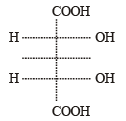(Plane of symmetry)

QUESTION: 21

CH3 – CHCl – CH2 – CH3 has a chiral centre. which one of the following represents its R-configuration? 

Solution: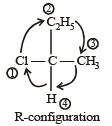QUESTION: 22

For (i) I, (ii) Cl, (iii) Br, the increasing order of nucleophilicity would be 

Solution:

Nucleophilicity increases down the periodic table.   I- > Br- > Cl- > F-

QUESTION: 23

The order of decreasing reactivity towards an electrophilic reagent, for the following:
i) Benzene
ii) Toluene
iii) Chlorobenzene
iv) Phenol

Solution:

Benzene having any activating group i.e, OH, R etc., undergoes electrophilic substitution very easily as compared to benzene itself. Thus toluene (C6H5CH3), phenol (C6H5OH) undergo electrophilic substitution very readily than benzene.Chlorine with +E and +M effect deactivating the ring due to strong -I effect. So, it is difficult to carry out the substitution  in chlorobenzene than in benzene, so correct order is

Phenol > Toluene > Benzene > Chlorobenzene

QUESTION: 24

Which one of the following is most reactive towards electrophilic attack ? 

Solution:

Out of the given compounds the most reactive towards nucleophilic attack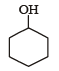Phenoxide ion is stable due to resonance. i.e. the correct answer is option (c).

QUESTION: 25

Base strength of :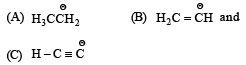is in the order of :

Solution:

The amount of s-character in various hybrid orbitals is as follows. sp = 50%, sp2 = 33% and sp3 = 25% Therefore character of the C – H bond in acetylene (sp) is greater than that of the C – H bond in alkene (sp2 hybridized) which in turn has greater s character of the C – H bond in alkanes. Thus  owing to a highs character of the C – H bond in alkynes, the electrons constituting this bond are more strongly held by the carbon nucleus with the result the hydrogen present on such a carbon atom can be easily removed as proton. The acidic nature of three types of C – H bonds follows the following order ≡ C-H > = C - H > - C - H.
Further, as we know that conjugate base of a strong acid is a weak base, hence the correct order of basicity is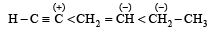QUESTION: 26

How many stereoisomers does this molecule have?

CH3CH = CHCH2CHBrCH3

Solution:

In the molecule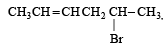the number of stereoisomers is given by sum of geometrical isomers (because of presence of C = C) and optical isomers (because of presence of chiral carbon atom).
Number of geometrical isomers = 2 (one C = C is present).
Number of optical isomers = 2 (one chiral carbon atom).
Total number of stereoisomers = 2 + 2 = 4

QUESTION: 27

A strong base can abstr act an α-hydrogen from :

Solution:

A strong base can abstract an α-hydrogen from a ketone.

QUESTION: 28

The stability of carbanions in the following :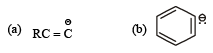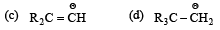is in the order of : 

Solution:

Higher the no. of electron releasing group lower will be stability of carbanion, and vice-versa. So the order of stability of carbanions is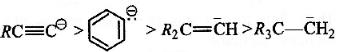QUESTION: 29

In the hydrocarbon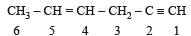The state of hybrization of carbons 1, 3 and 5 are in the following sequence : 

Solution:

C – 1 is sp hybridized (C ≡ C)
C – 3 is sp3 hybridized (C– C)
C – 5 is sp2 hybridized (C = C) Thus the correct sequence is sp, sp3, sp2.

QUESTION: 30

The state of hybridization of C2, C3, C5 and C6 of the hydrocarbon,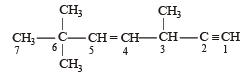is in the following sequence:

Solution: